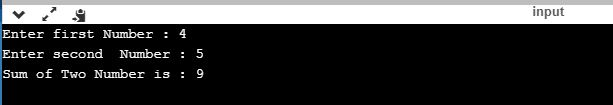UncleCoder.com

UncleCoder.com

Free programming examples and instructions

# C Program to find sum of two numbers using Function

## C Program for how to find sum of two numbers using Function

by Krishna

Posted on 27 Jul 2018 Category: C Views: 7254

C Program to find sum of two numbers using Function

In this program, user asks to find the sum of two numbers with use of function . The function calling procedure will use in this program to find the sum of two numbers. Here is source code of the C program that Find the sum of two numbers through function. The C program is successfully compiled. The program output is also shown below.

``````#include<stdio.h>
void main()
{
int a,b;

printf("Enter first Number : ");
scanf("%d",&a);
printf("Enter second  Number : ");
scanf("%d",&b);
sum(a,b);
getch();
}
sum(int x,int y)
{
int z;
z=x+y;
printf("Sum of Two Number is : %d",z);
return 0;
} ``````

OUTPUTRelated Articles

C Program to find sum of digits

C program to find sum of first 10 integers using for loop

C program to find largest among two numbers

C program to print Fibonacci series up to 100

C program to Check the number is Palindrome

C program to find Reverse of a Number

C program to find the factors of a number